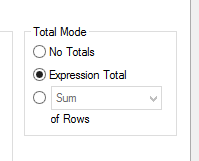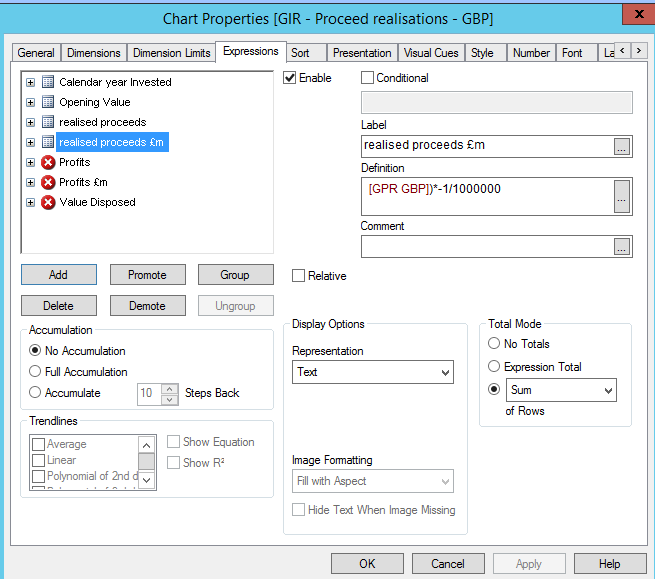Announcements
You can succeed best and quickest by helping others to succeed. Join the conversation.
cancel
Showing results for
Did you mean:Creator III

## Rounding using Floor/Ceil is there an alternative?

Hello

In an expression I am rounding to the nearest million by   /100000

Each individual row then appears correct to the nearest million but the total does not necessarily agree to the sum of the rounded individual lines.

I used the function floor, which works in the sense that the individual lines then always agree to the total but the logic of floor is wrong, ie £69,990,177 is rounded to £69m rather than £70m.

Is there anyway to have the individual lines rounded correctly ie to the nearest million but then also have the total agree to the individual lines?

Many thanks

Paul

1 Solution

Accepted SolutionsCreator II

Paul

I have used the following to round to the nearest ten (First line) I think what you would need is the second version

Floor(MyValue + 5, 10)

Floor(MyValue + 500000, 1000000)

Regards

5 RepliesMaster

Hi Paul,

Your best bet is only to round individual lines. If you're working with a straight table then in the Expressions tab set the Total Mode to Sum of Rows.

Cheers

AndrewCreator III

Hello ,

For total are you checking 'Sum of rows' then it will directly sum, else you can use 'expression total' it will calculte correctlyCreator III
Author

That didn't seem to work

You can see that the individual lines sum to 111, but the total says 112.  I would need it really to show 111expression

sum( {
<
entity -= {"0148"},
pl -={"X01*"}, proceeds = {"Proceeds"}, period = {">=\$(vStartPeriod_CY)<=\$(vEndPeriod_CY)"}
> }
[GPR GBP])*-1/1000000Creator II

Paul

I have used the following to round to the nearest ten (First line) I think what you would need is the second version

Floor(MyValue + 5, 10)

Floor(MyValue + 500000, 1000000)

Regards